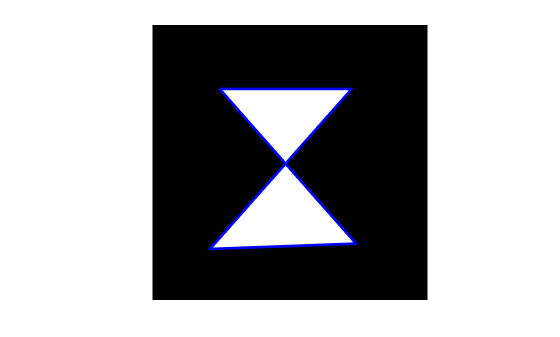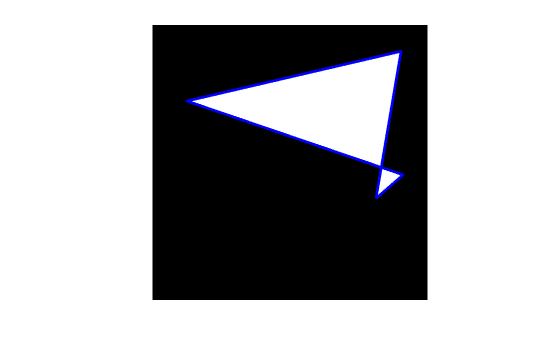Convert region of interest (ROI) polygon to region mask

## Syntax

``BW = poly2mask(xi,yi,m,n)``

## Description

example

````BW = poly2mask(xi,yi,m,n)` computes a binary region of interest (ROI) mask, `BW`, of size `m`-by-`n`, from an ROI polygon with vertices at coordinates `xi` and `yi`. If the polygon is not already closed, then `poly2mask` closes the polygon automatically.The `poly2mask` function sets pixels that are inside the polygon to `1` and sets pixels outside the polygon to `0`. For more information about classifying pixels on the ROI boundary, see Classify Pixels That Are Partially Enclosed by ROI.```

## Examples

collapse all

Specify the x- and y-coordinates of the polygon.

```x = [63 186 54 190 63]; y = [60 60 209 204 60];```

Create the mask specifying the size of the image.

`bw = poly2mask(x,y,256,256);`

Display the mask, drawing a line around the polygon.

```imshow(bw) hold on plot(x,y,'b','LineWidth',2) hold off```Define two sets of random points for the x- and y-coordinates.

```x = 256*rand(1,4); y = 256*rand(1,4); x(end+1) = x(1); y(end+1) = y(1);```

`bw = poly2mask(x,y,256,256);`

Display the mask and draw a line around the polygon.

```imshow(bw) hold on plot(x,y,'b','LineWidth',2) hold off```## Input Arguments

collapse all

x-coordinate of polygon vertices, specified as a numeric vector. The length of `xi` and `yi` must match.

Data Types: `double`

y-coordinate of polygon vertices, specified as a numeric vector. The length of `xi` and `yi` must match.

Data Types: `double`

Number of rows in the mask, specified as a nonnegative integer.

Data Types: `double`

Number of columns in the mask, specified as a nonnegative integer.

Data Types: `double`

## Output Arguments

collapse all

Binary image, returned as an `m`-by-`n` logical matrix.

Data Types: `logical`

## Tips

• To specify a polygon that includes a given rectangular set of pixels, make the edges of the polygon lie along the outside edges of the bounding pixels, instead of the center of the pixels.

For example, to include pixels in columns 4 through 10 and rows 4 through 10, you might specify the polygon vertices like this:

```x = [4 10 10 4 4]; y = [4 4 10 10 4]; mask = poly2mask(x,y,12,12)```
```mask = 0 0 0 0 0 0 0 0 0 0 0 0 0 0 0 0 0 0 0 0 0 0 0 0 0 0 0 0 0 0 0 0 0 0 0 0 0 0 0 0 0 0 0 0 0 0 0 0 0 0 0 0 1 1 1 1 1 1 0 0 0 0 0 0 1 1 1 1 1 1 0 0 0 0 0 0 1 1 1 1 1 1 0 0 0 0 0 0 1 1 1 1 1 1 0 0 0 0 0 0 1 1 1 1 1 1 0 0 0 0 0 0 1 1 1 1 1 1 0 0 0 0 0 0 0 0 0 0 0 0 0 0 0 0 0 0 0 0 0 0 0 0 0 0```

In this example, the polygon goes through the center of the bounding pixels, with the result that only some of the desired bounding pixels are determined to be inside the polygon (the pixels in row 4 and column 4 and not in the polygon). To include these elements in the polygon, use fractional values to specify the outside edge of the 4th row (3.5) and the 10th row (10.5), and the outside edge of the 4th column (3.5) and the outside edge of the 10th column (10.5) as vertices, as in the following example:

```x = [3.5 10.5 10.5 3.5 3.5]; y = [3.5 3.5 10.5 10.5 3.5]; mask = poly2mask(x,y,12,12)```
```mask = 0 0 0 0 0 0 0 0 0 0 0 0 0 0 0 0 0 0 0 0 0 0 0 0 0 0 0 0 0 0 0 0 0 0 0 0 0 0 0 1 1 1 1 1 1 1 0 0 0 0 0 1 1 1 1 1 1 1 0 0 0 0 0 1 1 1 1 1 1 1 0 0 0 0 0 1 1 1 1 1 1 1 0 0 0 0 0 1 1 1 1 1 1 1 0 0 0 0 0 1 1 1 1 1 1 1 0 0 0 0 0 1 1 1 1 1 1 1 0 0 0 0 0 0 0 0 0 0 0 0 0 0 0 0 0 0 0 0 0 0 0 0 0 0```

## Version History

Introduced before R2006a

expand all